R script: source1.R ; source2.R

$$\newcommand{\esp}{\mathbb{E}\left(#1\right)} \newcommand{\var}{\mbox{Var}\left(#1\right)} \newcommand{\deriv}{\dot{#1}(t)} \newcommand{\prob}{ \mathbb{P}\!(#1)} \newcommand{\eqdef}{\mathop{=}\limits^{\mathrm{def}}} \newcommand{\by}{\boldsymbol{y}} \newcommand{\bc}{\boldsymbol{c}} \newcommand{\bpsi}{\boldsymbol{\psi}} \def\pmacro{\texttt{p}} \def\like{{\cal L}} \def\llike{{\cal LL}} \def\logit{{\rm logit}} \def\probit{{\rm probit}} \def\one{{\rm 1\!I}} \def\iid{\mathop{\sim}_{\rm i.i.d.}} \def\simh0{\mathop{\sim}_{H_0}} \def\df{\texttt{df}} \def\res{e} \def\xomega{x} \newcommand{\argmin}{{\rm arg}\min_{#1}} \newcommand{\argmax}{{\rm arg}\max_{#1}} \newcommand{\Rset}{\mbox{\mathbb{R}}} \def\param{\theta} \def\setparam{\Theta} \def\xnew{x_{\rm new}} \def\fnew{f_{\rm new}} \def\ynew{y_{\rm new}} \def\nnew{n_{\rm new}} \def\enew{e_{\rm new}} \def\Xnew{X_{\rm new}} \def\hfnew{\widehat{\fnew}} \def\degree{m} \def\nbeta{d} \newcommand{\limite}{\mathop{\longrightarrow}\limits_{#1}} \def\ka{k{a}} \def\ska{k{\scriptscriptstyle a}} \def\kel{k{e}} \def\skel{k{\scriptscriptstyle e}} \def\cl{C{\small l}} \def\Tlag{T\hspace{-0.1em}{lag}} \def\sTlag{T\hspace{-0.07em}{\scriptscriptstyle lag}} \def\Tk{T\hspace{-0.1em}{k0}} \def\sTk{T\hspace{-0.07em}{\scriptscriptstyle k0}} \def\thalf{t{1/2}} \newcommand{\Dphi}{\partial_\pphi #1} \def\asigma{a} \def\pphi{\psi} \newcommand{\stheta}{{\theta^\star}} \newcommand{\htheta}{{\widehat{\theta}}}$$

# 1 Introduction

A system of ordinary differential equations defines a dynamical system. Then sources terms are inputs that modify the dynamics of the system by acting on the state variables, i.e on the components of the ODE system.

Source terms are defined in the R code, using the input argument treatment of simulx. Their action, i.e. the target variables, are defined either in the PK block of the Mlxtran code or in the R code itself, as an element of the input argument treatment.

In the case of a single type of source terms, treatment is a list with the following elements:

• amount : the values of the source terms. Can be a scalar or a vector if different values are used at different times.
• time : a vector of times.
• rate (or tinf) : constant input rate (or input duration = amount/rate). Can be a scalar or a vector (default rate = $$\infty$$, i.e. default duration = 0).
• type : type of input, when several inputs and targets are defined in the PK block of the Mlxtran code (default type = 1).
• target : name of the target component of the system (if not defined in the PK block).

Several types of source terms can be defined by defining treatment as a list of elementary source terms.

# 2 Examples

## 2.1 Example 1

We consider here the very simple following ODE system: $\deriv{h} = c$ with $$h(t)=0$$ for $$t\leq 0$$. Of course, the solution is a straight line with slope $$c$$, i.e. $$h(t) = c\,t$$

We modify this basic system by adding 2 source terms such that: \begin{align} h(30^+) &= h(30^-) - 2 \\ h(50^+) &= h(50^-) + 0.5 \end{align} (here, $$h(t^+)$$ is the value of $$h$$ just after $$t$$ and $$h(t^-)$$ the value just before $$t$$).

This model is implemented in source1a.txt: the ODE system is defined in the EQUATION block and the target is defined as $$h$$ in the PK block.

[LONGITUDINAL]
input={r}

PK:
depot(target=h)

EQUATION:
t0  = 0
h_0 = 0
ddt_h = r


We will use simulx for computing $$h$$ between $$t=0$$ and $$t=70$$ with $$r=0.1$$. The values and times of the inputs are defined in the list treatment:

tr <- list(amount = c(-2, 0.5),
time   = c(30, 50))

out  <- list(name = 'h',
time = seq(0, 70, by=0.1))

p    <- c( r = 0.1 )

res <- simulx(model='model/source1a.txt',
parameter=p,
output=out,
treatment=tr)

print(ggplot(data=resh) + geom_line(aes(x=time,y=h), colour="magenta" ,size=1))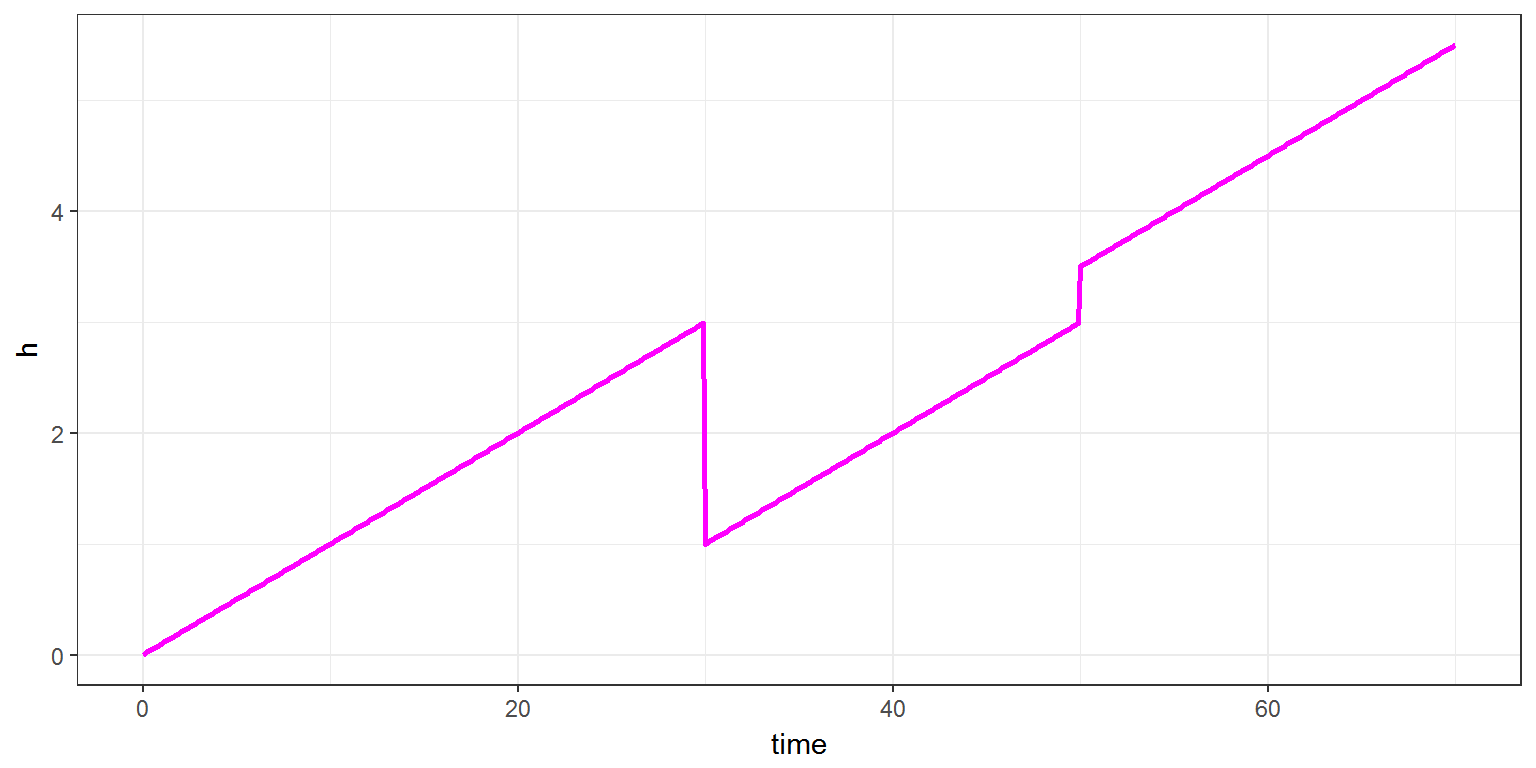Assume now that the state variable $$h$$ is not instantaneously modified, but modified with a constant rate $$r=0.5$$. The new model is therefore: \begin{align} \deriv{h} &= c \quad \text{for} \ \ t \leq 30 \\ \deriv{h} &= c - 0.5 \quad \text{for} \ \ 30 \leq t \leq 34 \\ \deriv{h} &= c \quad \text{for} \ \ 34 \leq t \leq 50 \\ \deriv{h} &= c + 0.5 \quad \text{for} \ \ 50 \leq t \leq 51 \\ \deriv{h} &= c \quad \text{for} \ \ t \geq 30 \end{align} The ODE system define in the Mlxtran code remains the same, but the source term are now defined by a rate $$r=0.5$$: tr <- list(amount = c(-2, 0.5), rate = 0.5, time = c(30, 50)) res <- simulx(model='model/source1a.txt', parameter=p, output=out, treatment=tr) print(ggplot(data=resh) + geom_line(aes(x=time,y=h), colour="magenta" ,size=1))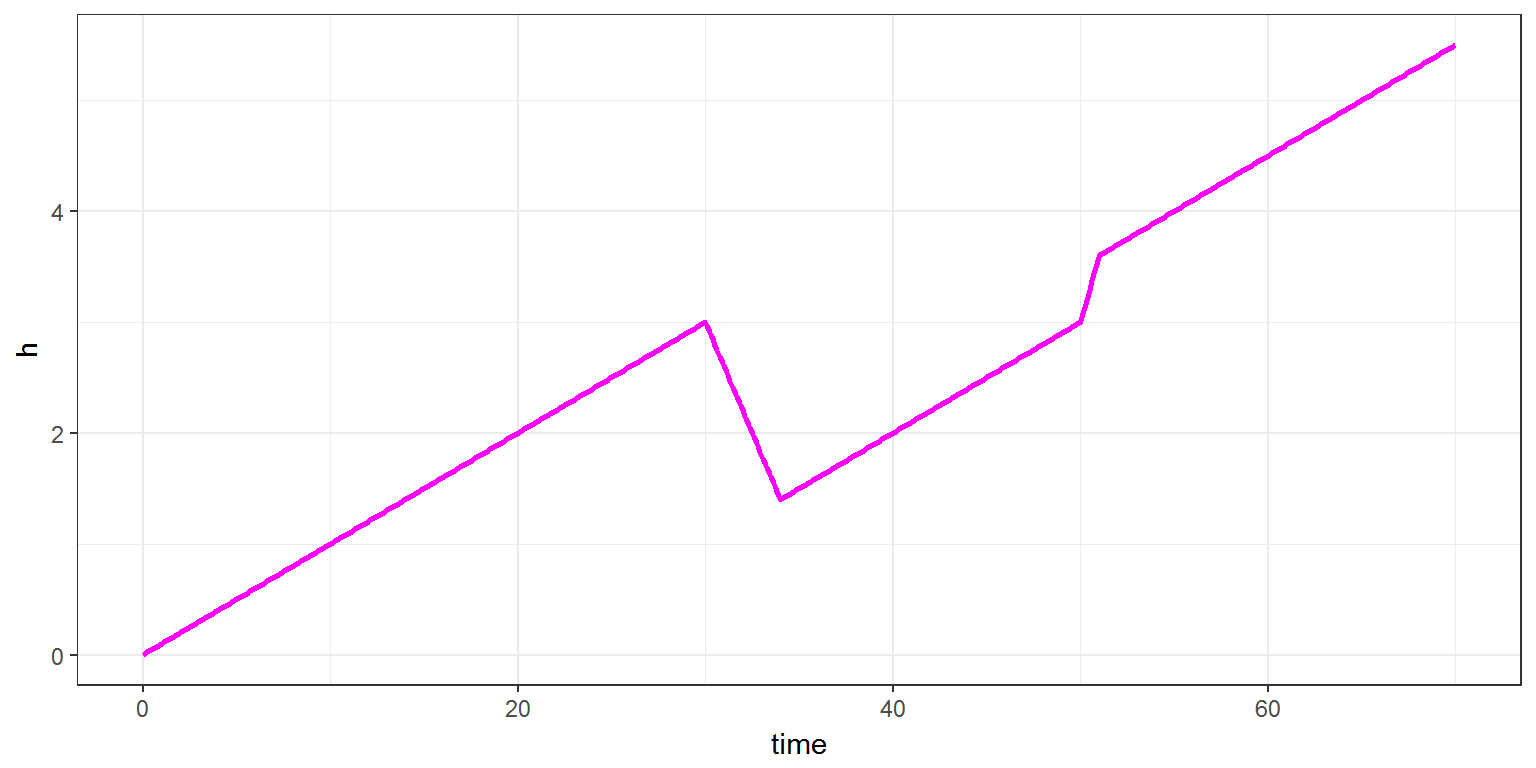Several source terms can be combined:

ton  <- list(amount = 1,
rate   = 1,
time   = c(5, 25))

toff <- list(amount = -1,
rate   = 0.25,
time   = c(10, 40))

res <- simulx(model='model/source1a.txt',
parameter=p,
output=out,
treatment=list(ton, toff))

print(ggplot(data=res$h) + geom_line(aes(x=time,y=h), colour="magenta", size=1))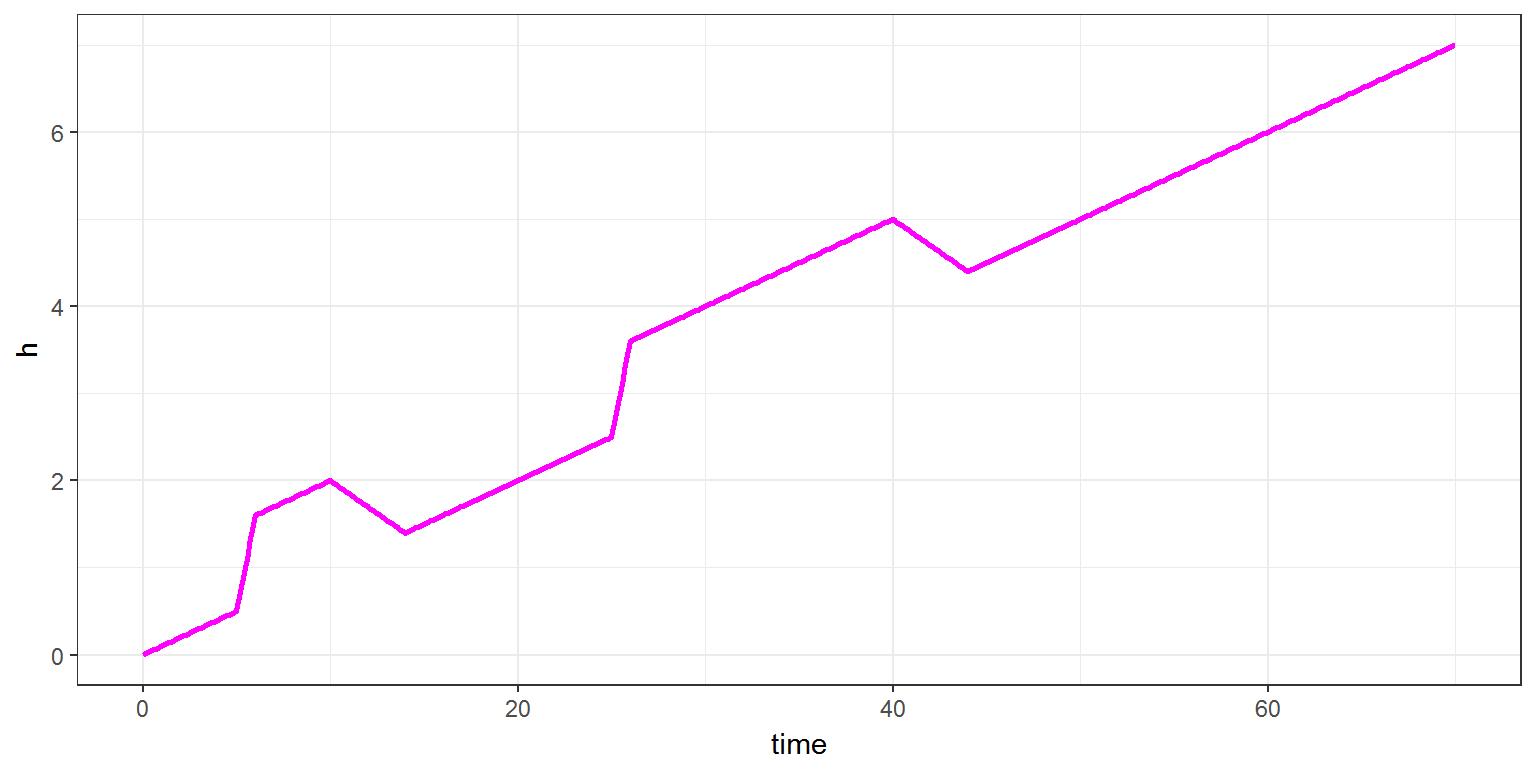Remark: Instead of defining 2 sequeneces of source terms (on and off), it would be equivalent to define a unique sequence of source terms: tr <- list(amount = c(1,-1,1,-1), rate = c(1,0.25,1,0.25), time = c(5, 10, 25, 40)) res <- simulx(model='model/source1a.txt', parameter=p, output=out, treatment=tr) print(ggplot(data=res$h) + geom_line(aes(x=time,y=h), colour="magenta", size=1))If a source term with value $$A_s$$ is added at time $$t_s$$, we can also assume that the source term acts with a delay $$\tau$$ and a factor $$F$$: \begin{align} h((t_s + \tau)^+) &= h((t_s+\tau)^-) + F\,A_s \end{align}

The delay $$\tau$$ and the factor $$F$$ are defined in the block PK of source2.txt as additional arguments Tlag and p of depot. Then, $$\tau$$ and $$F$$ are additional parameters of the model defined in the list input:

[LONGITUDINAL]
input={r, tau, F}

PK:
depot(target=h, Tlag=tau, p=F)

EQUATION:
t0  = 0
h_0 = 0
ddt_h = r


Values of $$\tau$$ and $$F$$ are defined in the simulx script:

p    <- c( r=0.1, tau=5, F=2 )

res <- simulx(model='model/source2.txt',
parameter=p,
output=out,
treatment=list(ton, toff))

print(ggplot(data=res$h) + geom_line(aes(x=time,y=h), colour="magenta", size=1))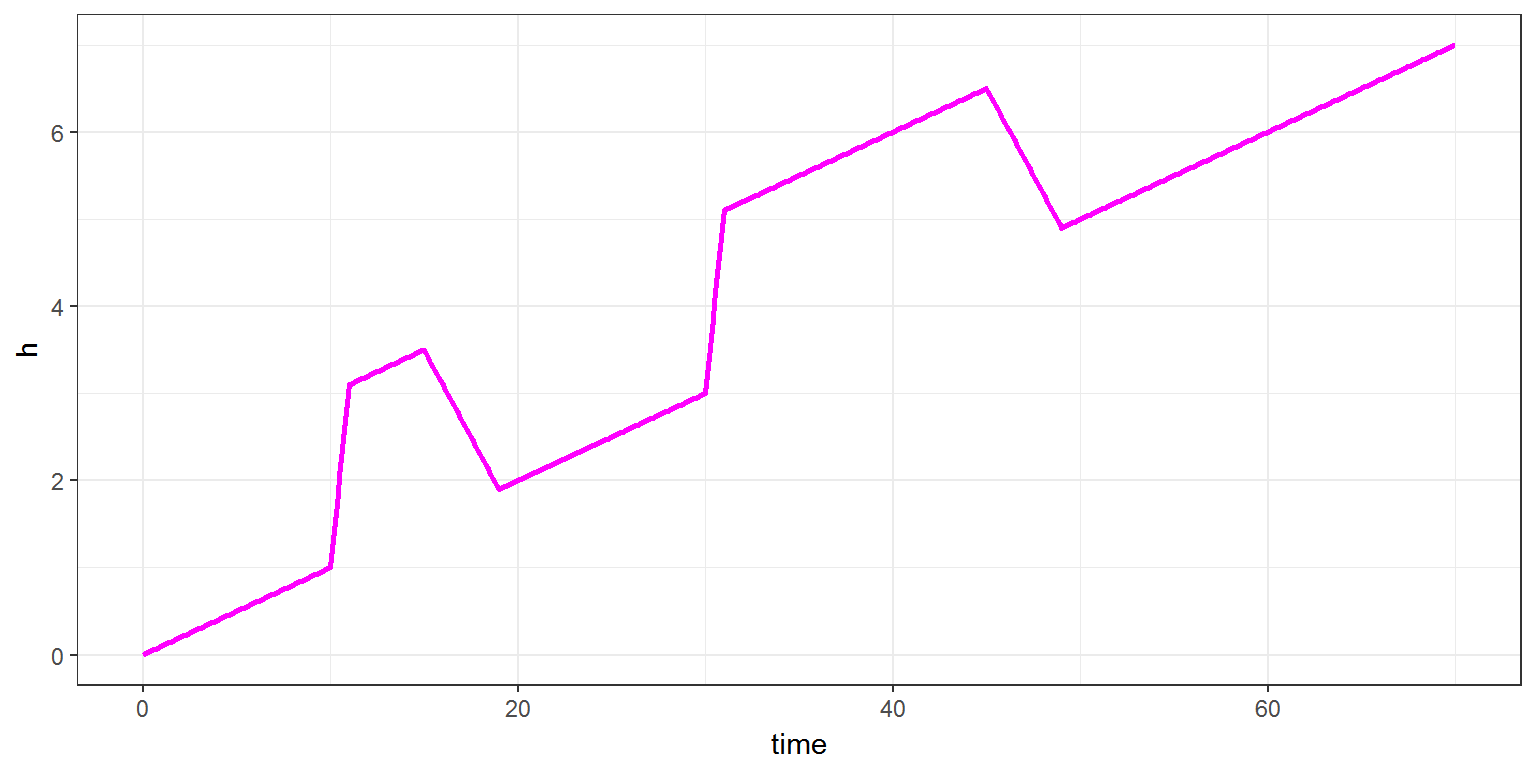Remark: It is possible to define the target component in the simulx script. Then, there is no more PK block in the Mlxtran code source1b.txt: [LONGITUDINAL] input={r} EQUATION: t0 = 0 h_0 = 0 ddt_h = r  tr <- list(amount = c(-2, 0.5), time = c(30, 50), target = 'h') res <- simulx(model='model/source1b.txt', parameter=p, output=out, treatment=tr) print(ggplot(data=res$h) + geom_line(aes(x=time,y=h), colour="magenta" ,size=1))## 2.2 Example 2

When the system consists of several variables, it is possible to define several source terms that act on different components of the system.

type now defines the type of each source term. A type is associated with a target component of the system.

An example with two types of source terms is implemented in source3a.txt. in this example, source terms of type 1 will act on $$f$$ while source terms of type 2 will act on $$g$$.

[LONGITUDINAL]
input = {k1, k2, f0, g0}

PK:
depot(type=1, target=f)
depot(type=2, target=g)

EQUATION:
t0  = 0
f_0 = f0
g_0 = g0
ddt_f = -k1*f + k2*g
ddt_g =  k1*f - k2*g


Each source term defined in the R script now has a type:

s1 <- list(type=1, time=c(10, 30, 40), amount=c(1,-1,1), rate=0.5)
s2 <- list(type=2, time=c( 5, 25, 35), amount=c(1,-0.5,1.5))

fg <- list(name=c('f','g'), time=seq(0, 50, by=0.1))

res <- simulx( model     = "model/source3a.txt",
parameter = c(k1=0.2, k2=0.1, f0=0, g0=0),
treatment = list(s1, s2),
output    = fg)

print(ggplot() + geom_line(data=res$f, aes(x=time, y=f, colour="blue")) + geom_line(data=res$g, aes(x=time, y=g, colour="red")) +
scale_colour_manual(name="",values=c('blue'='blue','red'='red'),labels=c('f','g')) +
theme(legend.position=c(0.9, 0.3)))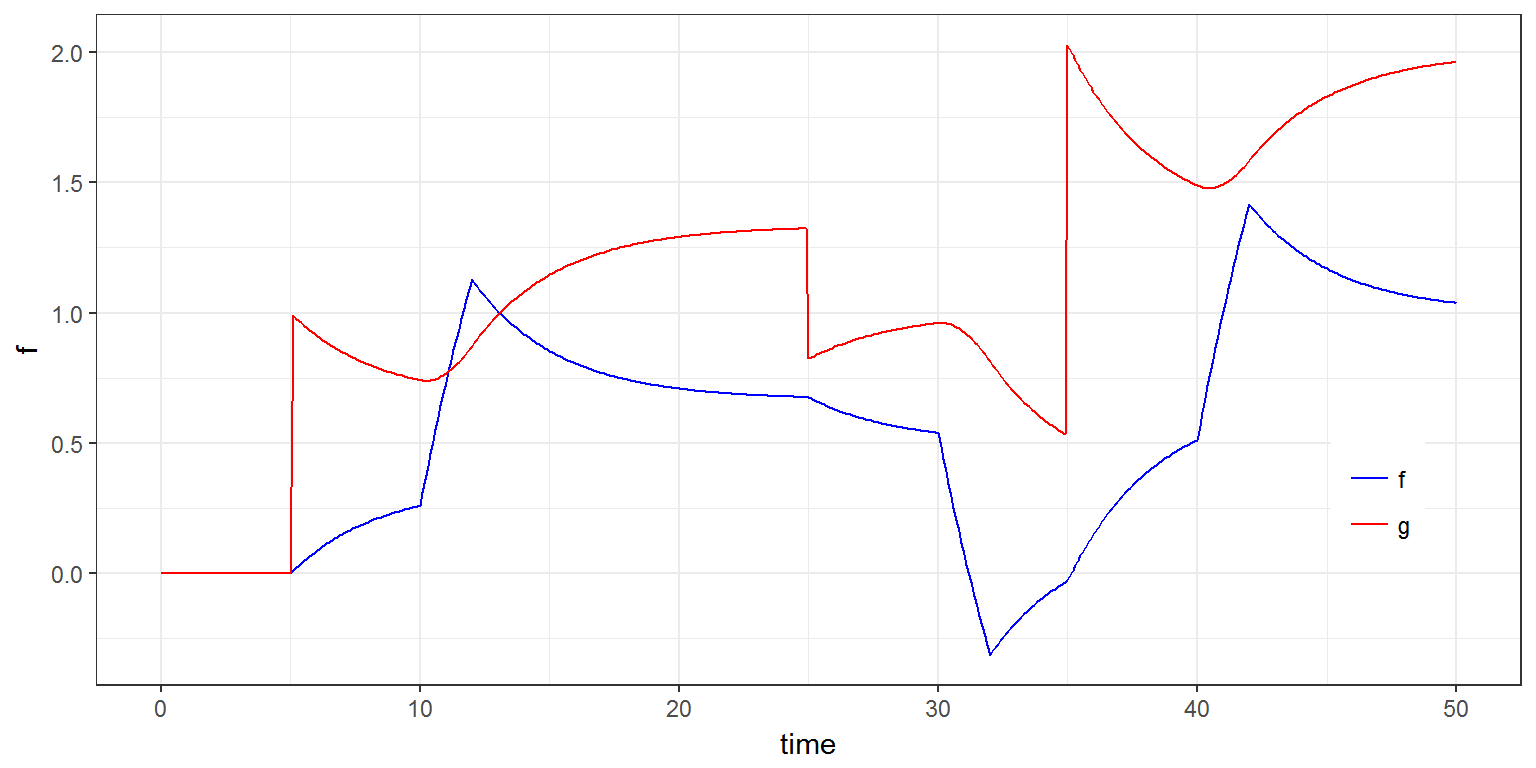Each source term may act with a delay and a multiplicative factor that can be function of the system itself.

Assume for instance that source terms of type 1 act on $$f$$ with a delay $$\tau$$ and that source term of type 2 at time $$t_s$$ act on $$g$$ with a multiplicatve factor $$f(t_s)/2$$. This new model is implemented in source4.txt.

[LONGITUDINAL]
input = {k1, k2, f0, g0, tau}

PK:
depot(type=1, target=f, Tlag=tau)
depot(type=2, target=g, p=f/2)

EQUATION:
t0  = 0
f_0 = f0
g_0 = g0
ddt_f = -k1*f + k2*g
ddt_g =  k1*f - k2*g

res <- simulx( model     = "model/source4.txt",
parameter = c(k1=0.2, k2=0.1, f0=0, g0=0, tau=2),
treatment = list(s1, s2),
output    = fg)

print(ggplot() + geom_line(data=res$f, aes(x=time, y=f, colour="blue")) + geom_line(data=res$g, aes(x=time, y=g, colour="red")) +
scale_colour_manual(name="",values=c('blue'='blue','red'='red'),labels=c('f','g')) +
theme(legend.position=c(0.9, 0.3)))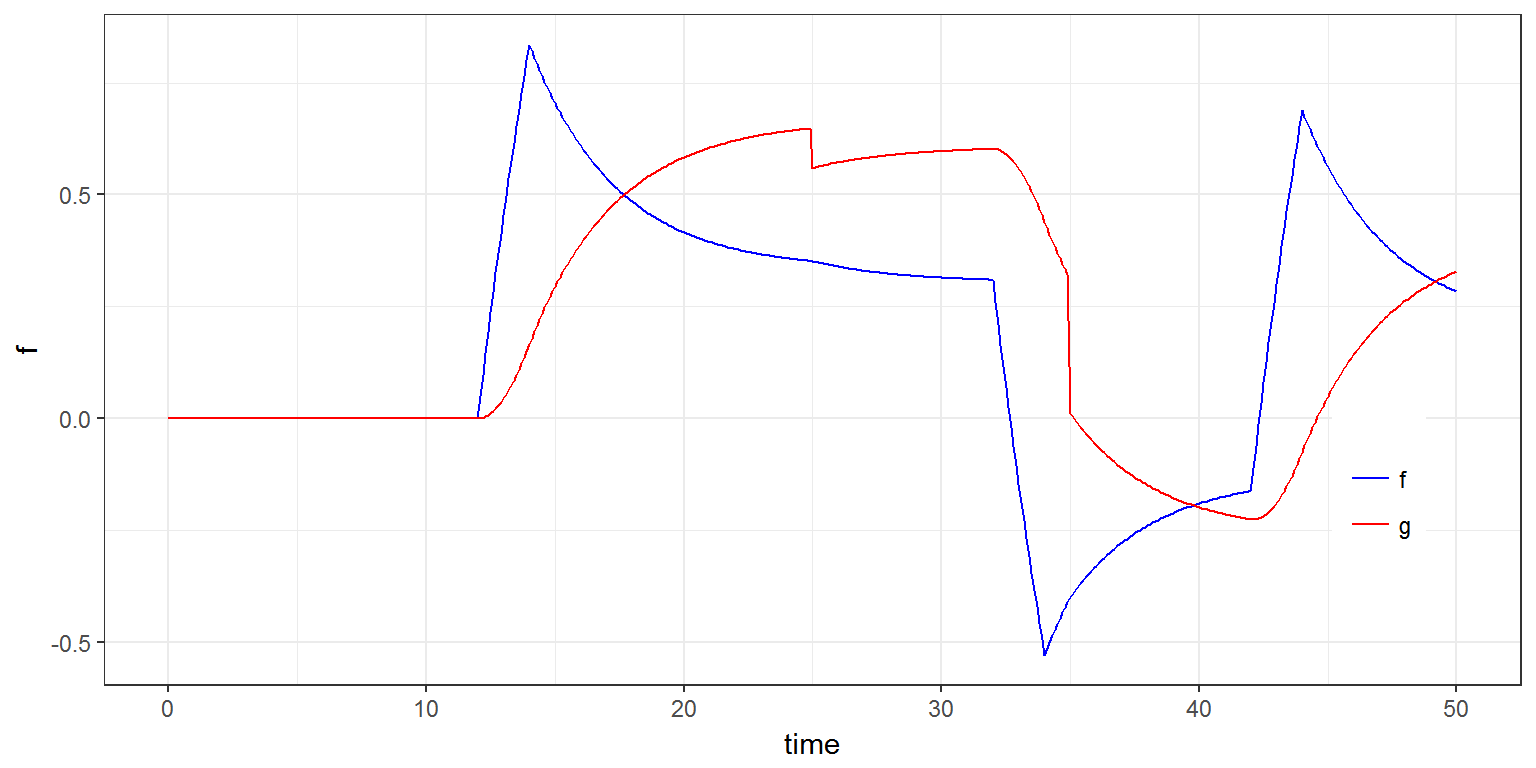Another version of model source3a.txt is implemented in source3b.txt. There is no PK block in this model and only the dynamical system is defined in the block EQUATION:

[LONGITUDINAL]
input = {k1, k2, f0, g0}

EQUATION:
t0  = 0
f_0 = f0
g_0 = g0
ddt_f = -k1*f + k2*g
ddt_g =  k1*f - k2*g


Then, both target components are defined with the input argument treatment of simulx:

s1 <- list(target="f", time=c(10, 30, 40), amount=c(1,-1,1), rate=0.5)
s2 <- list(target="g", time=c( 5, 25, 35), amount=c(1,-0.5,1.5))

res <- simulx( model     = "model/source3b.txt",
parameter = c(k1=0.2, k2=0.1, f0=0, g0=0),
treatment = list(s1, s2),
output    = fg)

print(ggplot() + geom_line(data=res$f, aes(x=time, y=f, colour="blue")) + geom_line(data=res$g, aes(x=time, y=g, colour="red")) +
scale_colour_manual(name="",values=c('blue'='blue','red'='red'),labels=c('f','g')) +
theme(legend.position=c(0.9, 0.3)))